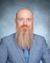# Get xy coordinates when a point is created

160
2
02-02-2023 09:26 AMOccasional Contributor

I have a client who wants to have the xy coordinates display in the popup window when a new point is collected. I have set up fields to hold the x and y coordinates. I've tried to write an expression to get the x coordinate and add the value to the x coordinate field and the same for the y coordinate field but have had no luck. Is this possible? If so, does someone know an expression that I could use to get the xy coordinates of a newly created point?

1 Solution

Accepted SolutionsOccasional Contributor

For all of those who were sitting on the edge of their seats waiting for a solution, here is the script to get the xy coordinates and display in decimal degrees:

```//To populate X coordinate in decimal

function MetersToLon(x) {

var originShift = 2.0 * PI * 6378137.0 / 2.0;

var lon = (x / originShift) * 180.0;

return Round(lon, 3);
}

return MetersToLon(Round(Geometry(\$feature).X, 6));```
```//To populate Y coordinate in decimal:

function MetersToLat(y) {

var originShift = 2.0 * PI * 6378137.0 / 2.0;

var lat = (y / originShift) * 180.0;

lat = 180.0 / PI * (2.0 * Atan( Exp( lat * PI / 180.0)) - PI / 2.0);
return Round(lat,3);
}
return MetersToLat(Round(Geometry(\$feature).Y, 6));Here is the article that I got the script from. Thanks esri support for helping me resolve this issue!How To: Calculate XY values using an Arcade expression in ArcGIS Pro (esri.com)```

2 RepliesOccasional Contributor

So I've been able to find an expression that gets the x and y coordinates and saves them to attribute fields with the following formula (edited as necessary for lat/long):

var geom = Geometry(\$feature)
if (!IsEmpty(geom)) {
return geom.x
} else {
return null
}

Unfortunately, the results are shown in meters and I need them in decimal degrees. Does anyone have a way to make this happen? From what I understand, it is not a straight-forward calc like converting pixels values from meters to feet in a raster. However, I may be wrong. Could someone please help me resolve this issue?Occasional Contributor

For all of those who were sitting on the edge of their seats waiting for a solution, here is the script to get the xy coordinates and display in decimal degrees:

```//To populate X coordinate in decimal

function MetersToLon(x) {

var originShift = 2.0 * PI * 6378137.0 / 2.0;

var lon = (x / originShift) * 180.0;

return Round(lon, 3);
}

return MetersToLon(Round(Geometry(\$feature).X, 6));```
```//To populate Y coordinate in decimal:

function MetersToLat(y) {

var originShift = 2.0 * PI * 6378137.0 / 2.0;

var lat = (y / originShift) * 180.0;

lat = 180.0 / PI * (2.0 * Atan( Exp( lat * PI / 180.0)) - PI / 2.0);
return Round(lat,3);
}
return MetersToLat(Round(Geometry(\$feature).Y, 6));Here is the article that I got the script from. Thanks esri support for helping me resolve this issue!How To: Calculate XY values using an Arcade expression in ArcGIS Pro (esri.com)```